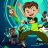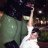# McGinley Dynamic Indicator for ThinkorSwim#### BenTen

Staff
VIP
Warehouse
This indicator is called McGinley Dynamic for ThinkorSwim. Investopedia dubbed it "The Most Reliable Indicator You've Never Heard Of". I thought it would be interesting to share it here.

The tradition simple and exponential moving averages tend to lag. McGinley Dynamic indicator will try to overcome that by automatically adjusting itself relative to the speed of the market.### thinkScript Code

Code:
``````# McGinley Dynamic Indicator
# Mobius
# V02.11.2011
#  D = D1 + ((I - D1) / ( N * (I/D1)^4))
# where D1= yesterday's Dynamic, I = today's price, N = smoothing factor.

input value= close;
input length= 20;
input Slength = 5;
def A1= ExpAverage(value, length);
def MDI = A1 + ((value - A1) / Sqr(value / A1));
plot Data = ExpAverage(MDI, Slength);
Data.SetPaintingStrategy(PaintingStrategy.LINE);
Data.SetLineWeight(2);
#Data.SetDefaultColor(GetColor(8));
def A2 = ExpAverage(value, length*3);
def MDI2 = A2 + ((value - A2) / Sqr(value / A2));
plot Data2 = ExpAverage(MDI2, Slength*3);
Data2.DefineColor("Data2_SlopePos", Color.GREEN);
Data2.DefineColor("Data2_SlopeNeg", Color.MAGENTA);
Data2.AssignValueColor(if Data  > Data2 then Data2.Color("Data2_SlopePos") else Data2.Color("Data2_SlopeNeg"));
Data2.setlineweight(2);
data2.hide();
Data.DefineColor("Data_SlopePos", Color.CYAN);
Data.DefineColor("Data_SlopeNeg", Color.RED);
Data.AssignValueColor(if Data  > Data2 then Data.Color("Data_SlopePos") else Data.Color("Data_SlopeNeg"));
data.hide();
plot EMA8 = expaverage(close,8);
EMA8.setdefaultcolor(color.DARK_ORANGE);
EMA8.setlineweight(2);
EMA8.hide();
# End Code ------------
def buySignal = if Data > Data2 and Data <= Data2 and expaverage(close,8) > average(close,13) then 1 else 0;
def sellSignal = if Data < Data2 and Data >= Data2 and expaverage(close,85) < average(close,13) then 1 else 0;
plot signal = if buySignal or sellSignal then Data else double.nan;
signal.assignValueColor(if buySignal then color.WHITE else color.YELLOW);
signal.setLineWeight(5);
#signal.SetStyle(curve.points);
signal.setpaintingstrategy(paintingStrategy.SQUARES);

# additional plots from yahoo forum
input price = close;
input length2 = 14;
input displace = 0;
plot WS = WildersAverage(price[-displace], length2);
WS.setDefaultColor(GetColor(8));
input periods = 10;
rec _md = compoundValue( 1, _md + (( close - _md ) / ( periods *
power( close / _md, 4 ) ) ), close );
plot MD = _md;
MD.setdefaultcolor(color.CYAN);
MD.setlineweight(2);
MD.hide();

def buySignal2 = if MD > WS and MD <= WS and expaverage(close,8) > average(close,13) then 1 else 0;
def sellSignal2 = if MD < WS and MD >= WS and expaverage(close,85) < average(close,13) then 1 else 0;
plot signal2 = if buySignal2 or sellSignal2 then MD else double.nan;
signal2.assignValueColor(if buySignal2 then color.WHITE else color.YELLOW);
signal2.setLineWeight(5);
signal2.SetStyle(curve.points);
#signal2.setpaintingstrategy(paintingStrategy.SQUARES);``````
Here is the simplified version that paints your candles to the trend of the indicator.

Code:
``````#McGinleyIndicator
declare upper;

input periods = 10;

rec _md = CompoundValue( 1, _md + (( close - _md ) / ( periods * Power( close / _md, 4 ) ) ), close );

plot MD = _md;
AssignPriceColor(if MD > MD then Color.GREEN else Color.RED);``````

Credits:

•NDN3531 and mc01439#### mc01439

##### Active member
VIP
Warehouse
This indicator seems to give much more information for a better trade with the following plots. It seems the other plots were added to the McGinley Dynamic Indicator but I think they enhance it.

Data - ON
Date2 - ON
EMA8 - OFF
Signal - ON
WS - OFF
MD - OFF
Signal2 - OFF because seems to be a late or confirming signal

Tried to paste a chart image but not sure how to use the new insert image.

Thank You for posting.

•BenTen#### mc01439

##### Active member
VIP
Warehouse
Is the (close,85) in the code a mistake, shouldn't it be 8? The same with signal 2

def buySignal = if Data > Data2 and Data <= Data2 and expaverage(close,8) > average(close,13) then 1 else 0; def sellSignal = if Data < Data2 and Data >= Data2 and expaverage(close,85) < average(close,13) then 1 else 0;#### BenTen

Staff
VIP
Warehouse
@mc01439 It could’ve been. I didn’t notice. Do you see any difference when correcting it?#### mc01439

##### Active member
VIP
Warehouse
Yes it does. Ran a strategy test on day charts for 5 years. This is a raw run - signal to signal.

For Signal
set at 85 (\$66170) 28 trades
set at 8 (\$310) 28 trades

For Signal 2
set at 85 +\$4530 18 trades
set at 8 (\$3130) 17 trades#### mc01439

##### Active member
VIP
Warehouse
Ran a strategy test on Weekly for 10 years. This is a raw run - signal to signal.

For Signal
set at 85 (\$39680) 18 trades
set at 8 (\$55240) 16 trades#### BenTen

Staff
VIP
Warehouse
Hmm. Interesting. Why is it that Signal 2 (set to 85) is profitable?#### mc01439

##### Active member
VIP
Warehouse
Hmm. Interesting. Why is it that Signal 2 (set to 85) is profitable?

I will need to look deeper. Did the test above real quick.#### mc01439

##### Active member
VIP
Warehouse
Another option written by Mobius.

This one tested out signal to signal on the 5 year Daily @ \$73,640.00. Please keep in mind this is a raw test.

Code used for the test;
def HT = MDI > MDI;
def LT = MDI < MDI;

# Archive Name: McGinley Dynamic Indicator_Mobius
# Archive Section: Trend
# Suggested Tos Name: McGinleyDynamicIndicator_Mobius
# Archive Date: 5.05.2018
# Archive Notes: Lounge 5.02.2018
# 16:59 Mobius: An oldie but goodie
# 17:01 Mobius: The lag on that is only a few bars and that lag can be mostly removed by detrending it by half the input length

# McGinley Dynamic Indicator
# Mobius
# V03.11.2011
# D = D + (I - D) / ( N * (I/D)^4)
# where D = yesterday's Dynamic, I = today's price, N = smoothing factor.

input I = close;
input N = 13;

def D;
D = CompoundValue(1, D + (I - D) / (N * Power((I / D), 4)), I);

plot MDI = D;
MDI.SetPaintingStrategy(PaintingStrategy.LINE);
MDI.SetLineWeight(1);
MDI.SetDefaultColor(Color.White);

# End Code McGinley Dynamic Indicator

•markos
C

#### cherif

##### Member
The close,85 is only a typo. Close,8 is the correct code entry.

•mc01439 and BenTenIndicators 3Questions 7